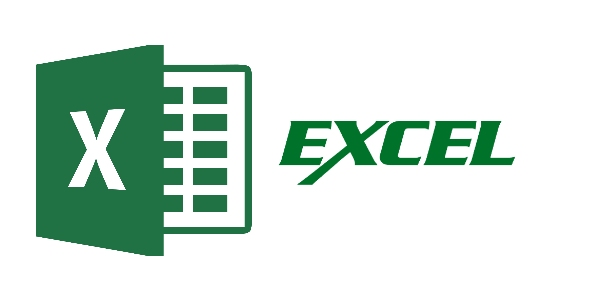# Excel

20 Questions | Total Attempts: 203SettingsTake an exam to see how well you know some Excel basics. Content in this quiz was excerpted from Training on Microsoft Office Online.

Related Topics
• 1.
• A.

Click Subtotals on the Data menu.

• B.

View the sum in the formula bar.

• C.

Click the AutoSum button on the Standard toolbar, then press ENTER.

• 2.
• A.

Click the Paste button on the Standard toolbar.

• B.

Click the arrow on the Paste button on the Standard toolbar, then click Formulas.

• C.

Click the arrow on the Paste button on the Standard toolbar, then click Values.

• 3.
How do you change column width to fit the contents?
• A.

Single-click the boundary to the left of the column heading.

• B.

Double-click the boundary to the right of the column heading.

• C.

Press ALT and single-click anywhere in the column.

• 4.
There are three worksheets with every new workbook. You can change that automatic number if you want to.
• A.

True

• B.

False

• 5.
###### means:
• A.

You've entered a number wrong.

• B.

You've misspelled something.

• C.

The cell is not wide enough.

• 6.
To add a new row, click a cell in the row immediately above where you want the new row.
• A.

True

• B.

False

• 7.
Which key do you press to group two or more nonadjacent worksheets?
• A.

CTRL.

• B.

SHIFT

• C.

ALT

• 8.
To copy an entire worksheet and all its data, you should click the worksheet tab of the sheet that you want to copy, hold down SHIFT, and then drag the selected sheet along the row of sheet tabs.
• A.

True

• B.

False

• 9.
• A.

Go to FILE - SAVE AS - SAVE AS TYPE - Excel 4.0 Work Sheet

• B.

Right click on the spreadsheet tab and select DELETE

• C.

Right click on the spreadsheet and select INSERT - ENTIRE COLUMN

• 10.
Which formula can add the all the numeric values in a range of cells, ignoring those which are not numeric, and place the result in a different cell ?
• A.

Count

• B.

Average

• C.

Sum

• 11.
Is it possible to insert an image from a file into an Excel spreadsheet ?
• A.

Yes

• B.

No

• 12.
Can an Excel spreadsheet be used as the "data source" for a Word Mail Merge ?
• A.

Yes

• B.

No

• 13.
On an Excel sheet the active cell is indicated by ____.
• A.

A dark wide border

• B.

A dotted border

• C.

• D.

None of the above

• 14.
A Formula and a function are the same thing.
• A.

True

• B.

False

• 15.
In order to multiply items in Excel you would use:
• A.

^

• B.

@

• C.

*

• D.

#

• 16.
The formula = ((A2+B5)*5% is valid
• A.

True

• B.

False

• 17.
If cells: A1=90 A2=85 A3=80 A4=75 A5=75 What will be your formula if you are going to get the average?
• 18.
If cells: A1=90 A2=85 A3=80 A4=75 A5=75 What will be your formula if you are going to get the total?
• 19.
Get the remarks in cell E10 that valued 75 which noted, if the remarks will be higher than 74, the remarks will be "PASSED" or else "FAILED".
• 20.
Get the remarks in cell A1 that valued 75 which noted, if the remarks will be higher than 74, the remarks will be "PASSED" or else "FAILED".